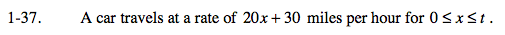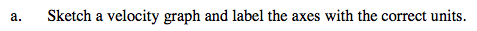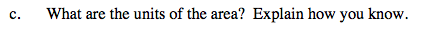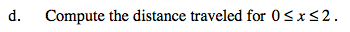### Home > CALC > Chapter 1 > Lesson 1.2.2 > Problem1-37

1-37.The area represents distance traveled (or displacement) between x = 0 and x = t.The shape is a trapezoid.

$\text{base_1 and base_2 are vertical, so they represent velocity \left(in\ \frac{miles}{hour}\right).}$

Height is horizontal, so it represents time, in hours. Evaluate the area of a trapezoid. Simplify the units.Find A (20x + 30, 0 ≤ x ≤ 2).

Use the eTool below to set values for t.
Click the link at right for the full version of the eTool: Calc 1-37 HW eTool